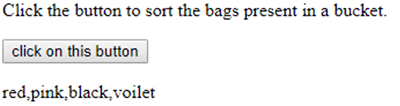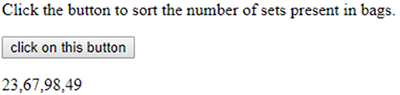JavaScript Sort Function - Coding Tag# Sort Function in JavaScript

2114

Hi there,

I hope you are familiar with Arrays? If not, you can go through the previous topics of this tutorial. In this blog, I will highlight the enhancement of the Array usefulness which includes sorting through Sort function.

The first question that may arise in our mind through this topic is that "what is sorting".

Let's begin with sorting.

The process of organizing the items systematically is known as sorting. This sorting plays a prime role while categorizing certain items with identical properties. Certainly, when items are arranged in sequence wise in the system through programming language /algorithms is known as the sorting process.

In JavaScript, there are several algorithms or functions that are meant to perform sort operations. In all those sorting functions, the Sort function is one of them.

### JavaScript Sort Function

sort() function is used in JavaScript to perform the sorting of an Array containing elements in it. However, the original arrays are being modified through sort function. Basically, when there is a need to sort array in alphabetical order, then this function has been implemented by developers.

Below mentioned is the syntax used for sort ():

`Array.sort([comparisonFunction])`

The above-given syntax includes an optional argument. The main objective of this argument is to accomplish a comparison between two elements present in an Array.

Whenever the compare function is absent in the syntax then the sort function will operate normally i.e. depending upon the values of elements, sorting will be performed.

In JavaScript, while using elements values in a Sort function, there are various protocols used for implementing comparison functions. Some of them are mentioned below:

If comparisonFunction, if (p,q) is less than 0, then p will come at the initial position.

If comparisonFunction, if (p,q) is > than (greater than 0) ,then q will come at initial position.

If comparisonFunction, if (p,q) is 0 then the positions of both p and q remain unchanged.

This sort() consents two arguments which further returns some values.

For instance, consider the following small code containing comparison operators:

```function ballsInBucket.sort(p,q){
If (p>q) {
Return 1;
Else if (q>p){
Return-1;
}
Else{
Return 0;
}
}```

Real time use case of Sorting:

Suppose we will get a group of balls in a bucket, then the task will be assigned to us to perform sorting in both ascending and descending. How we will do it?

It is very Simple. Sort() function can be used

For example:

`ballsInBucket.sort();`

Sort Function Program:

```<!DOCTYPE html>
<html>
<body>

<p>Click the button to sort the bags present in a bucket.</p>
<button onclick="myFunction()">click on this button</button>
<p id="demo"></p>

<script>
var ballsInBucket = ["red", "pink", "black", "voilet"];
document.getElementById("demo").innerHTML = ballsInBucket;
function myFunction() {
ballsInBucket.sort();
ballsInBucket.sort();
document.getElementById("demo").innerHTML = ballsInBucket;
}
</script>
</body>
</html>```

Output:On Clicking on "click on this button" this output will be displayed the sorted element in alaphabetic order. This is illustrated through the following output:A simple program illustrating sorting of numerical values:

```<!DOCTYPE html>
<html>
<body>

<p>Click the button to sort the number of sets present in bags.</p>
<button onclick="myFunction()">click on this button</button>
<p id="demo"></p>

<script>
var ballsInBucket = ["23", "67", "98", "49"];
document.getElementById("demo").innerHTML = ballsInBucket;
function myFunction() {
ballsInBucket.sort();
ballsInBucket.sort();
document.getElementById("demo").innerHTML = ballsInBucket;
}
</script>
</body>
</html>```

Output:The output appears after clicking on a buttonShare:## Get a .COM for just \$6.98

Secure Domain for a Mini Price